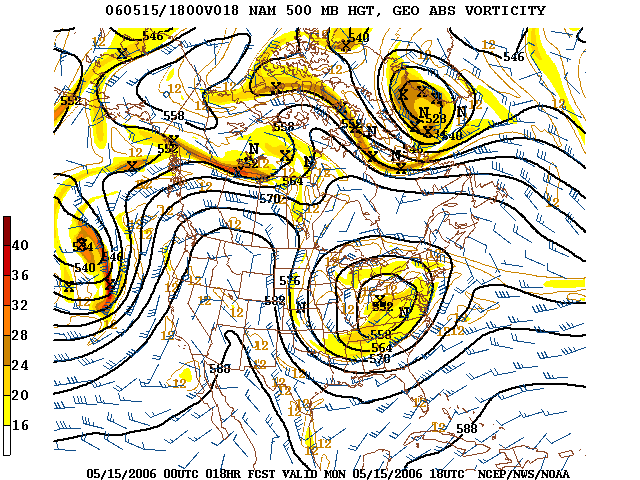$$\newcommand{\id}{\mathrm{id}}$$ $$\newcommand{\Span}{\mathrm{span}}$$ $$\newcommand{\kernel}{\mathrm{null}\,}$$ $$\newcommand{\range}{\mathrm{range}\,}$$ $$\newcommand{\RealPart}{\mathrm{Re}}$$ $$\newcommand{\ImaginaryPart}{\mathrm{Im}}$$ $$\newcommand{\Argument}{\mathrm{Arg}}$$ $$\newcommand{\norm}{\| #1 \|}$$ $$\newcommand{\inner}{\langle #1, #2 \rangle}$$ $$\newcommand{\Span}{\mathrm{span}}$$

# 6.6: Putting It Together- Factoring

$$\newcommand{\vecs}{\overset { \rightharpoonup} {\mathbf{#1}} }$$ $$\newcommand{\vecd}{\overset{-\!-\!\rightharpoonup}{\vphantom{a}\smash {#1}}}$$$$\newcommand{\id}{\mathrm{id}}$$ $$\newcommand{\Span}{\mathrm{span}}$$ $$\newcommand{\kernel}{\mathrm{null}\,}$$ $$\newcommand{\range}{\mathrm{range}\,}$$ $$\newcommand{\RealPart}{\mathrm{Re}}$$ $$\newcommand{\ImaginaryPart}{\mathrm{Im}}$$ $$\newcommand{\Argument}{\mathrm{Arg}}$$ $$\newcommand{\norm}{\| #1 \|}$$ $$\newcommand{\inner}{\langle #1, #2 \rangle}$$ $$\newcommand{\Span}{\mathrm{span}}$$ $$\newcommand{\id}{\mathrm{id}}$$ $$\newcommand{\Span}{\mathrm{span}}$$ $$\newcommand{\kernel}{\mathrm{null}\,}$$ $$\newcommand{\range}{\mathrm{range}\,}$$ $$\newcommand{\RealPart}{\mathrm{Re}}$$ $$\newcommand{\ImaginaryPart}{\mathrm{Im}}$$ $$\newcommand{\Argument}{\mathrm{Arg}}$$ $$\newcommand{\norm}{\| #1 \|}$$ $$\newcommand{\inner}{\langle #1, #2 \rangle}$$ $$\newcommand{\Span}{\mathrm{span}}$$

Factoring polynomials may seem like a tedious task that is put forth only to cause you frustration. Try to think of factoring like running. When we do it, we become fit, even though we may feel at first as if we are going to pass out. The algebra involved in factoring helps your brain make connections about multiplication and division and how they are related to each other. This makes you more mathematically fit. Like a trained runner, being mathematically fit means you can accomplish impressive feats.But even the best human athletes are no competition for machines. In the 1960s theoretical physicists and researchers interested in artificial intelligence (AI) began to develop computer algebra systems, which can quickly “out-math” a human.

A computer algebra system (CAS) is a software program that allows computation of mathematical expressions in a way which is similar to the traditional manual computations of mathematicians and scientists. The development of the computer algebra systems in the second half of the 20th century is part of the discipline of “computer algebra” or “symbolic computation”, which has spurred work in algorithms over mathematical objects such as polynomials.

There have been many advocates for increasing the use of computer algebra systems in primary and secondary school classrooms in the form of an AI agent, which is kind of like a part-human, part-computer teacher. Interestingly, the use of CAS in the classroom not only supports students as they learn, but can also help teachers improve the way they teach because computer algebra systems emphasize the process of learning.In addition to the software of CAS, polynomials can be used to graph curves, which makes them ideal for designing roller coasters. We can also use polynomials to better understand the stock market by using polynomials to see how prices change over time, to trace the projectile of objects in physics, and even to predict weather trends in meteorology.